# Everything of Similar Triangle: Notes, Problems and SolutionsSimilar Triangles

Whenever we talk about two congruent figures then they have the ‘same shape’ and the ‘same size’. There are figures that are of the ‘same shape but not necessarily of the ‘same size’. They are said to be similar. Congruent figures are similar but the converse is not true

All regular polygons of same number of sides are similar. They are equilateral triangles, squares etc. All circles are also similar.

Two polygons of the same number of sides are similar if their corresponding angles are sides are proportional.

Two triangles are similar if their corresponding are equal and corresponding sides are proportional.

Basic Proportionality Theorem or Thales Theorem.

Theorem-1

If a line is drawn parallel to one side of a triangle, to interest the other two sides indistinct points, the other two sides are divided in the same ratio.

Given: – In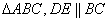To prove:-Construction:- BE and CD are joined.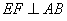and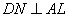are drawn.

Proof:-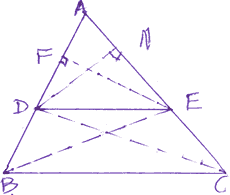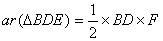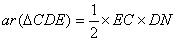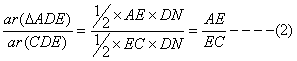But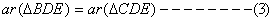as they are on the same base DE and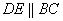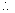from (1), (2) and (3) we getCorollary: InthenProof:- We know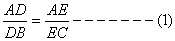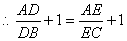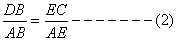(Taking reciprocals)

Multiplying (1) and (2) we get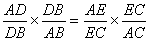Property – 1. If a line divides any two sides of a triangle in the same ratio, the line is parallel to the third side.

Example 1. In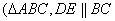andFind AB.

Solution:-(given)(Thales Theoram)= 3.6cm

= 2.4 + 3.6

= 6.0cm

Example 2. In the given figure,and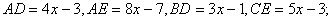Find x.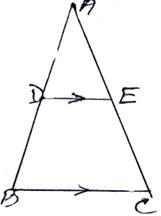Solution:-

In(given)Or, (4x – 3) (5x – 3) = (8x – 7) (3x – 1)

•  20x2 – 12x – 15x + 9 = 24x2 – 8x – 21x + 7
•  4x2 – 2x – 2 = 0
• 2x2 – x – 1 = 0
• 2x2 – 2x + x – 1 = 0
• 2x(x – 1) + 1 (x – 1) = 0
• (x – 1) (2x + 1) = 0
• x = 1, -1/2

But sides of a triangle cannot be negative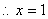Example 3.ABCD is a trapezium such thatIts diagonals AC and BD intersest each other at o.

prove that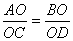.

Solution:- Given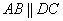AC and BD intersectato

To Prove: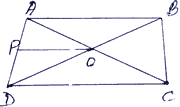Construction: OP || AB || CD is drawn

Proof:- InIn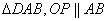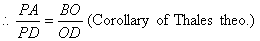From (i) and (ii) we getExample 4. Prove that any line parallel to parallel sides of a trapezium divides the non-parallel sides proportionally (i.e. in the same ratio)

Solution:-

Given:- PQRS is a trapezium in which PQ || SR || XY

To Prove:-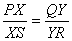Construction:- PR is joined which intersects XY at A.

Proof:- In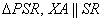[ Thales Theorem]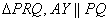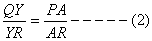( Taking reciprocals)

From (i) and (ii) we get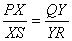Example 5. In the given figure DE || AQ and DF || AR

Prove that EF || QR

Solution:-

In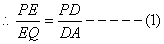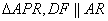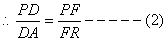from (1) and (2) we get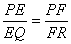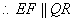### Exercise

1. In the given figure, PQ || BC, AP = 2.4cm, AQ = 2cm, QC = 3cm and BC = 6cm. Find AB and PQ.2. The diagonals AC and BD of a quadrilatereal ABCD intersect each other at O such that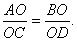prove that the quadrilateral ABCD is traperzium.

3. In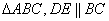andif AC = 4.8cm, find AE

4. In the given figurer, PQ || BC and PR || CD,

prove that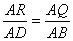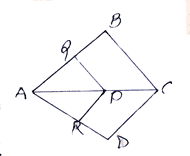5. Inis parallel to base BC, with D on AB and E on AC. If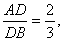find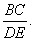6. In the given figure, PQ || AB and PR || AC. prove that QR || BC.7. If three or more parallel lines, are intersected by two transversals, prove that the intercepts made by them on the trans versals are proportional.

8. In the given figure, DE || AC and DC || AP, prove that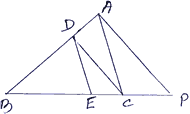9. In the given figure,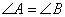and DE || AB prove that AD = BE.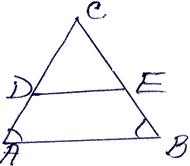10. In the given figure AB || CD. If OA = 3x – 19, OB = x – 4, OC = x – 3 and OD = 4cm, determine x.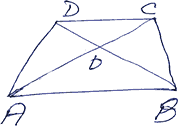Answers (10). (x = 11 cm or 8 cm)
Don't miss out!